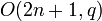# There are at most two finite simple groups of any order

## Statement

Let$n$ be a natural number. Then, there are at most two finite simple groups of order$n$.

The smallest value of$n$ for which there are two non-isomorphic simple groups of order$n$ is$20160 = 2^6 \cdot 3^2 \cdot 5 \cdot 7$. The two groups in this case are the alternating group of degree eight (which is also isomorphic to the projective special linear group$PSL(4,2)$) and projective special linear group:PSL(3,4).

## Description of relevant pairs

### Infinite families

Note that there are examples other than the infinite families given below.

Below,$q = p^r$ is a prime power denoting the size of the field over which we are considering stuff.

Condition on$q$ Condition on$n$ First family (Chevalley notation) First family (description) Second family (Chevalley notation) Second family (description) Order
odd (when$q$ is even, the corresponding groups are in fact isomorphic)$n > 2$ (for$n = 1$ or$n = 2$, the corresponding groups are in fact isomorphic)$B_n(q)$ Chevalley group of type B, more explicitly this is$\Omega_{2n+1}(q)$, the intersection of kernels of the Dickson invariant and spinor norm in the orthogonal group$O(2n+1,q)$$C_n(q)$ projective symplectic group$PSp(2n,q)$$\! q^{n^2} \left[\prod_{i=1}^n (q^{2i} - 1)\right]/\operatorname{gcd}(2,q-1)$

### First few examples

These include both the infinite families and the examples not arising from infinite families.

Order First group Second group
20160 alternating group:A8 (isomorphic to$PSL(4,2)$) projective special linear group:PSL(3,4)
4585351680 Chevalley group of type B:B3(3) projective symplectic group:PSp(6,3)

## Proof

All known proofs of this fact employ the classification of finite simple groups, along with some explicit computations.